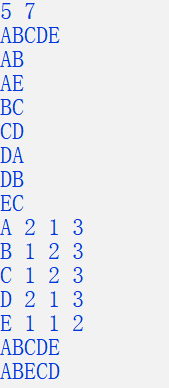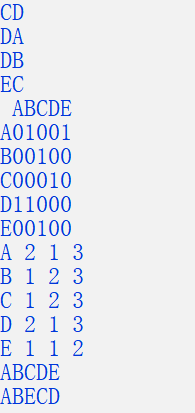• //输出有向图的领接矩阵 Calulate_Degree(G);//算度 TraverseG(G);//深度优先遍历 printf("\n"); TraverseG_BFS(G); //算度 测试 /* int n=0; int w=4; printf("%d %c\n",First_Adjmatrim_VEX(G,n),...
#include<stdio.h>
#include<malloc.h>
#include<string.h>
#define MAXVEX 20  //最大顶点个数
#define INFINTY -1  //表示无穷
//∞无穷
typedef struct{
int arcs[MAXVEX][MAXVEX];//边(弧)信息
char vex[MAXVEX];  //顶点信息
int vexnum;//顶点数目
int arcnum;//边（弧）数目

typedef struct {
int in;
int out;
int total;
}Degree[MAXVEX];

typedef struct{
int vex[MAXVEX];
int front;
int rear;
}*QUEQUE,QUEQUE_1;

int visited[MAXVEX]={0};

QUEQUE Create_QUEQUE();//创建队列
int Empty(QUEQUE Q);//队列判空
void out_QUEQUE(QUEQUE Q,int *w);//出队
void input_QUEQUE(QUEQUE Q,int w);//入队

main()
{
Create(G);
Calulate_Degree(G);//算度
TraverseG(G);//深度优先遍历
printf("\n");
TraverseG_BFS(G);

//算度 测试
/*
int n=0;
int w=4;
*/
}

{
return G;
}

{
int i,j,k,weight;
char vex1,vex2;
scanf("%d %d",&G->vexnum,&G->arcnum);//输入顶点数目和边数目

for(i=0;i<MAXVEX;i++)
for(j=0;j<MAXVEX;j++)
G->arcs[i][j]=-1;  //初始化

char  G_vexnum[G->vexnum];
scanf("%s",G_vexnum);

for(i=0;i<G->vexnum;i++)
{
G->vex[i]=G_vexnum[i]; // 初始化G->vexnum个结点

}

for(i=G->vexnum;i<MAXVEX;i++) //其它结点的信息初始化为NULL
{
G->vex[i]=NULL;

}

char vex1_vex2;
for(k=0;k<G->arcnum;k++)
{
scanf("%s",vex1_vex2);
vex1=vex1_vex2;
vex2=vex1_vex2;
i=Locate(G,vex1);
j=Locate(G,vex2);
G->arcs[i][j]=1;
}

}

{
int i;
for(i=0;i<G->vexnum;i++)
if(G->vex[i]==v)
return i;
return 0;
}

{
int i,j;
printf(" ");
for(i=0;i<G->vexnum;i++)
printf("%c",G->vex[i]);

printf("\n");
for(i=0;i<G->vexnum;i++)
{
printf("%c",G->vex[i]);
for(j=0;j<G->vexnum;j++)
{
if(G->arcs[i][j]==-1)//空
printf("0");
else
printf("%d",G->arcs[i][j]);
}
printf("\n");
}

}

{
int i,j;
Degree A;
int indext;
for(i=0;i<G->vexnum;i++)//算入度
{
indext=0;
for(j=0;j<G->vexnum;j++)
if(G->arcs[i][j]==1)
{
indext++;
}
A[i].out=indext;
}

for(i=0;i<G->vexnum;i++)//算出度
{
indext=0;
for(j=0;j<G->vexnum;j++)
if(G->arcs[j][i]==1)
{
indext++;
}
A[i].in=indext;
}

for(i=0;i<G->vexnum;i++)//算总度
{
A[i].total=A[i].in+A[i].out;
}

for(i=0;i<G->vexnum;i++)
printf("%c %d %d %d\n",G->vex[i],A[i].out,A[i].in,A[i].total);
}

{
printf("%c",G->vex[n]);
visited[n]=1;
while(w!=-1)
{
if(!visited[w])
DFS(G,w);
}
}

{
int i;
for(i=0;i<MAXVEX;i++)
visited[i]=0;
for(i=0;i<G->vexnum;i++)
if(!visited[i])
DFS(G,i);
}

{
int i;
int flag=-1;
for(i=w+1;i<G->vexnum;i++)
if(G->arcs[n][i]!=-1 )//
{
flag=i;break;
}
//	printf("Next %d\n",flag);
return flag;

}

{
int i;
for(i=0;i<G->vexnum;i++)
{
if(G->arcs[n][i]!=-1)
{
//			printf("First %d\n",i);
return i;
}
}
}
/*
{
int w,v;
QUEQUE Q=Create_QUEQUE();
if(v0<G->vexnum)
printf("%c",G->vex[v0]);
visited[v0]=1;
input_QUEQUE(Q,v0);
while(!Empty(QUEQUE(Q)))
{
out_QUEQUE(Q,&v);
while(w!=-1)
{
if(visited[w]!=1)
{
if(w<G->vexnum)
printf("%c",G->vex[w]);
visited[w]=1;
input_QUEQUE(Q,w);
}
}
}
}
*/
{
QUEQUE Q=Create_QUEQUE();
printf("%c",G->vex[v0]);
visited[v0]=1;
input_QUEQUE(Q,v0);
while(!Empty(Q))
{
int v;
out_QUEQUE(Q,&v);
while(w!=-1)
{
if(visited[w]!=1)
{
printf("%c",G->vex[w]);
visited[w]=1;
input_QUEQUE(Q,w);
}
}
}
}

{
int i;
for(i=0;i<MAXVEX;i++)
visited[i]=0;
for(i=0;i<G->vexnum;i++)
if(!visited[i])
BFS(G,i);
}

void out_QUEQUE(QUEQUE Q,int *w)
{
if(!Empty(Q))
{
*w=Q->vex[Q->rear];
Q->rear--;
}
}

void input_QUEQUE(QUEQUE Q,int w)
{
Q->rear++;
Q->vex[Q->rear]=w;
}

int Empty(QUEQUE Q)
{
if(Q->rear==-1)
return 1;
else
return 0;
}

QUEQUE Create_QUEQUE()
{
QUEQUE Q=(QUEQUE)malloc(sizeof(QUEQUE_1));
Q->front=Q->rear=-1;
return Q;
}

/*
5 7
ABCDE
AB
AE
BC
CD
DA
DB
EC

*/

运行结果：

不输出领接矩阵输出领接矩阵展开全文• 代码还是要求严格输入操作，没有判错能力。...void Interrupt(void)//创建一个中断函数 { while(1)//用于检测换行符，使函数脱离scanf连续输出 if(getchar()=='\n') break; } typedef struct.代码还是要求严格的输入操作，没有判错能力。见谅！
代码如下：
#include <stdio.h>
#include <stdlib.h>
#include <string.h>
#define MAX_VERTEX_NUM 20

void Interrupt(void)//创建一个中断函数
{
while(1)//用于检测换行符，使函数脱离scanf的连续输出
if(getchar()=='\n')
break;
}

typedef struct ArcBox
{
int weight;//权值
}ArcBox;
typedef struct VexNode
{
char data;//弧的名称
ArcBox *firstin,*firstout;//分别指向该顶点第一条入弧和出弧
}VexNode;
typedef struct
{
VexNode xlist[MAX_VERTEX_NUM];//表头向量
int vexnum,arcnum;//有向图的当前顶点数和弧数
}OLGraph;

void CreateGraph(OLGraph &G)//图的创建
{
int i;//记录次数
printf("请输入顶点数和边数: ");
scanf("%d %d",&G.vexnum,&G.arcnum);//顶点数和边数的赋值
Interrupt();//该函数用于检测并吸收换行符
for(i=0;i<G.vexnum;i++)//图的初始化，放在给定顶点之后可省去步骤
{
G.xlist[i].firstin = NULL;
G.xlist[i].firstout = NULL;
}
printf("请输入顶点名称（连续输入）：");
for(i=0;i<G.vexnum;i++)//利用循环输入图中顶点名称
scanf("%c",&G.xlist[i].data);//第i个顶点的命名
Interrupt();//该函数用于检测并吸收换行符
char b,c;//顶点变量
int w,j,k,l;//w为权值变量，j和k是用来记录次数的
ArcBox *p1,*p2;//创建两个野结点
for(i=0;i<G.arcnum;i++)//利用循环输入所有边的两个顶点和权值
{
printf("请输入边的两个顶点和权值w:");
scanf("%c %c %d",&b,&c,&w);//输入
Interrupt();//该函数用于检测并吸收换行符
for(j=0;j<G.vexnum;j++)//该操作为书上的函数LocateVex操作
{
if(G.xlist[j].data == b)//找到输入的顶点b的位置
break;
}
for(k=0;k<G.vexnum;k++)
{
if(G.xlist[k].data == c)//找到输入的顶点c的位置
break;
}
//出弧操作
p1 = (ArcBox*)malloc(sizeof(ArcBox));//申请空间
p1->tailvex = k;//尾位置和头位置的赋值
p1->weight = w;//权值赋值
G.xlist[j].firstout = p1;	//并使头结点永远放在第一位
//入弧操作
if(G.xlist[k].firstin == NULL)
G.xlist[k].firstin = p1;
else
{
p2 = G.xlist[k].firstin;
}
}
}

void InputGraph(OLGraph G)//十字链表的输出
{
int i,j;//记录次数
ArcBox *p;//用于遍历链表
printf("十字链表为：\n");
//为了能验证创建的图是否正确，分别输出顶点的入弧和出弧
//出弧
for(i=0;i<G.vexnum;i++)//利用循环输出
{
printf("%c",G.xlist[i].data);
p = G.xlist[i].firstout;
while(p)//当p为空时，结束循环
{
}
printf("\n");
}
//入弧
for(i=0;i<G.vexnum;i++)//利用循环输出
{
printf("%c",G.xlist[i].data);
p = G.xlist[i].firstin;
while(p)//当p为空时，结束循环
{
}
printf("\n");
}
}

int main()
{
OLGraph G;
CreateGraph(G);//图的创建
InputGraph(G);//图的输出
return 0;
}

代码结果演示：展开全文数据结构 链表 十字链表
• *顺序储存有向图邻接矩阵 *定义一个边权值二维数组 *定义一个点一维数组 *创建一个邻接矩阵 *输出这个邻接矩阵 */ #include<stdio.h> #include<string.h> #include<stdlib.h> #define ...
/*
*顺序储存有向图邻接矩阵
*定义一个边的权值的二维数组
*定义一个点的一维数组
*创建一个邻接矩阵
*输出这个邻接矩阵
*/
#include<stdio.h>
#include<string.h>
#include<stdlib.h>
#define MAXVERTEX 100//顶点数
#define ERROR 0
#define OK 1
typedef   char * VertexType;//顶点数据类型
typedef int ArcType;//权值数据类型
typedef int Statu;//返回值的数据类型
typedef enum{

DG,//有向图
UDG,//无向图
DN,//有向网
UDN//无向网
}GraphKind;//图的枚举类型
typedef struct
{
VertexType vertex[MAXVERTEX];//点的一维数组
ArcType arcs[MAXVERTEX][MAXVERTEX];//权值的二维数组
int vertexcount;//点数
int arccount;//边数
GraphKind kind;//图的类型
}Mtrix_Graph;//图的结构体类型
Statu create_matrixgraph(Mtrix_Graph*G);//创建邻接矩阵
void test();//测试函数
int locate_graph(Mtrix_Graph*G,VertexType vex);//返回名称在数组中的下标（定位函数）
void main()
{
test();//测试函数

}
Statu create_matrixgraph(Mtrix_Graph*G)//创建邻接矩阵
{
G->kind=UDG;//表明是无向图  （UDG代表有向图）
int i;
int j;
int x,y;
printf("输入图的顶点数:");
scanf("%d",&G->vertexcount);
printf("输入图的边数:");
scanf("%d",&G->arccount);
printf("请依次输入顶点的信息:\n");
for( i=0;i<G->vertexcount;i++)
{
G->vertex[i]=(VertexType)malloc(sizeof(char)*10);//分配内存空间
printf("顶点%d：",i+1);
scanf("%s",G->vertex[i]);
}
for(i=0;i<G->vertexcount;i++)//邻接矩阵初始化
for(j=0;j<G->vertexcount;j++)
G->arcs[i][j]=0;
//输入边的顶点位置 创建邻接矩阵
for( i=0;i<G->arccount;i++)
{
VertexType vex1=(VertexType)malloc(sizeof(char)*10);
//创建临时字符串变量
VertexType vex2=(VertexType)malloc(sizeof(char)*10);
printf("顶点：");
scanf("%s",vex1);
printf("临接点：");
scanf("%s",vex2);
x=locate_graph(G,vex1);
y=locate_graph(G,vex2);
if(x==-1||y==-1)
return ERROR;
else{
G->arcs[x][y]=1;
//G->arcs[y][x]=G->arcs[x][y];//无向图对称原因
free(vex1);//临时变量释放空间
free(vex2);
}
}
return OK;
}
int  locate_graph(Mtrix_Graph*G,VertexType vex)//返回名称在数组中的下标
{
int index=0;
while(index<G->vertexcount)
{
if(strcmp(vex,G->vertex[index])==0)
{
break;
}
else
index++;
}
return index==G->vertexcount?-1:index;

}
void test()//测试函数
{
Mtrix_Graph *G;
int i,j;
G=(Mtrix_Graph*)malloc(sizeof(Mtrix_Graph));
Statu result =create_matrixgraph(G);
if(result==ERROR)
{
printf("创建邻接矩阵失败:\n");
return ;
}
printf("打印一个邻接矩阵:\n");
printf("\t");//格式控制最上面的一行字母和矩阵数字对齐
for(i=0;i<G->vertexcount;i++)
{
printf("\t%s",G->vertex[i]);//输出第一行的字母

}
printf("\n");//第一行字母打印完之后换行
for(i=0;i<G->vertexcount;i++)
{
printf("\t%s",G->vertex[i]);//输出每一行的第一个字母 \t 缩进八个字节
for(j=0;j<G->vertexcount;j++)
printf("\t%d",G->arcs[i][j]);//缩进八个字节
printf("\n");//输入每一行之后换行
}

}




展开全文• 图的创建，以及利用图的几种不同的存储方法而实现的不同的创建方法，对无向图，有向图，无向网，以及有向网都与很好的说明，是你学习数据结构时必不可少的好东西.,,,,
• 这里有点懵！ 还是按书上的例子（这里用邻接...#define MVNum 100 //图的最大容量 ，也可以称为图的最大顶点数 void Interrupt(void)//创建一个中断函数 { while(1)//用于检测换行符，使函数脱离scanf的连续输出
这里有点懵！
还是按书上的例子（这里用邻接矩阵表示）完整代码如下：
#include <stdio.h>
#include <stdlib.h>
#include <string.h>
#define MaxInt 32767//无穷值设置
#define MVNum 100 //图的最大容量 ，也可以称为图的最大顶点数

void Interrupt(void)//创建一个中断函数
{
while(1)//用于检测换行符，使函数脱离scanf的连续输出
if(getchar()=='\n')
break;
}

typedef struct//图的结构体
{
char vexs[MVNum];//顶点表
int arcs[MVNum][MVNum];//邻接矩阵
int vexnum,arcnum;//图的当前点数和边数
}AMGraph;

void InitGraph(AMGraph &G)//图的初始化
{
int i,j;
for(i = 0;i<MVNum;i++)
for(j = 0;j<MVNum;j++)
G.arcs[i][j] = MaxInt;//使邻接矩阵中的数据都初始化为0
}

void CreateGraph(AMGraph &G)//图的创建
{
int i;//记录次数
char a;//顶点变量
printf("请输入顶点数和边数:");
scanf("%d %d",&G.vexnum,&G.arcnum);
Interrupt();//该函数用于检测并吸收换行符
printf("请输入顶点名称（连续输入）：");
for(i=0;i<G.vexnum;i++)
{
scanf("%c",&a);
G.vexs[i] = a;//第i个顶点的命名
}
Interrupt();//该函数用于检测并吸收换行符
char b,c;//顶点变量
int w,j,k;//w为权值变量，j和k是用来记录次数的
for(i=0;i<G.arcnum;i++)
{
printf("请输入边的两个顶点和权值w:");
scanf("%c %c %d",&b,&c,&w);//输入
Interrupt();//该函数用于检测并吸收换行符
for(j=0;j<G.vexnum;j++)
{
if(G.vexs[j] == b)//找到输入的顶点b的位置
break;
}
for(k=0;k<G.vexnum;k++)
{
if(G.vexs[k] == c)//找到输入的顶点c的位置
break;
}
G.arcs[j][k] = w;//权值赋值
}
}

void InputGraph(AMGraph G)//邻接矩阵的输出
{
int i,j;//记录次数
printf("邻接矩阵为：\n   ");
for(i=0;i<G.vexnum;i++)//打印顶点名称
printf("%c  ",G.vexs[i]);
printf("\n");
for(i=0;i<G.vexnum;i++)
{
printf("%c  ",G.vexs[i]);//打印顶点名称
for(j=0;j<G.vexnum;j++)//遍历邻接矩阵
printf("%d  ",G.arcs[i][j]);
printf("\n");
}
printf("\n");
}

void ShortestPath_Floyd(AMGraph G)//用Floyd算法求有向网G中各对顶点i和j之间的最短路径
{
int D[G.vexnum][G.vexnum],//定义辅助数组D[][]
Path[G.vexnum][G.vexnum];//定义辅助数组Path[][]
int i,j,k;//记录次数变量
for(i=0;i<G.vexnum;i++)//各对结点之间初始已知路径及距离
for(j=0;j<G.vexnum;j++)
{
D[i][j] = G.arcs[i][j];
if(i == j)
D[i][j] = 0;
if(D[i][j]<MaxInt && i!=j)//如果i和j之间有弧，则将j的前驱置为i
Path[i][j] = i;
else//如果i和j之间无弧，则将j的前驱置为-1
Path[i][j] = -1;
}
for(k=0;k<G.vexnum;k++)
for(i=0;i<G.vexnum;i++)
for(j=0;j<G.vexnum;j++)
if(D[i][k]+D[k][j]<D[i][j])//从i经k到j的一条路径最短
{
D[i][j] = D[i][k]+D[k][j];//更新D[i][j]
Path[i][j] = Path[k][j];//更改j的前驱为k
}
//下面可以省略
for(i=0;i<G.vexnum;i++)//为了验证是否正确，输出辅助数组Path最后的值
{
printf("Path[%d]",i);
for(j=0;j<G.vexnum;j++)
printf("   %d",Path[i][j]);
printf("\n");
}

}

int main()
{
AMGraph G;
InitGraph(G);//图的初始化
CreateGraph(G);//创建邻接矩阵
InputGraph(G);//输出邻接矩阵
ShortestPath_Floyd(G);//Floyd算法
return 0;
}

过程图：结果演示：（完）


展开全文数据结构 最短路径
• C语言设计对二叉树和有向图存储和运算算法程序 ...（4）创建由输入文件input.txt指定随机字母（结点个数不小于10个）的有向图，设计并实现非递归的有向图广度优先遍历算法程序，遍历结果输出到指定文件output.txtc 算法 设计
• 对无向图的每个顶点vi建立一个单链表，第i个单链表中的结点表示依附于顶点vi的边（对于有向图则是以顶点vi为尾的弧）。这个单链表就称为顶点vi的边表（对于有向图则称为出边表） 边表的头指针和顶点的数据信息采用...数据结构 单链表
• 简介： 该程序主要是用C语言来实现与图相关的算法，目的在于加深对于图...1.根据有向图的邻接矩阵创建该图的邻接表; 2.打印有向图的邻接表; 3.销毁一个图的邻接表; 4.有向图的邻接表的深度优先遍历和广度优先...
• 无向图创建邻接矩阵、深度优先遍历和广度...如果E中顶点对是有序，即E中每条边都是有方向，则称G是有向图。如果顶点对是无序，则称G是无向图 （2）邻接矩阵： 邻接矩阵主要由：二维数组 实现 如图 转数据结构 算法
• 数据结构整理-图的创建-求度-深度优先遍历-广度优先遍历图的创建定义邻接矩阵的数据类型用邻接矩阵创建图求有向图的出度，入度求出度求入度图的遍历深度优先遍历广度优先遍历测试数据及其结果总体代码如下 图的创建 ...数据结构 算法 队列 dfs
• 用邻接矩阵法创建一个有向网，将有向边直接视为无向边后，得到对应向图，则利用Prim算法求取最小生成树MST；利用Dijkstra算法对无向图求取顶点V1对图中其余各点最短路径。（c语言） ![图片说明]...
• 有向图：在有向图中，顶点对<V,W>是有序，表示它从V到W一条有向边。所以，<W,V>表示和<V,W>不同边，顶点指向不同。有向图中顶点对用尖括号表示<>，<x,y>数据结构 链表 单链表 指针
• 与图有关的信息主要有顶点信息和边（或弧度）的信息，图的分为有向图和无向图（方向指两个顶点的边的方向），以下是一个简单的...有向图的创建、求度、遍历（用领接矩阵存储法） 完整代码： #include<stdio.h...
• 答案是肯定，就是把它们整合在一起、这就是有向图一种存储方法：十字链表 定义顶点结构如图1-1所示。 图1-1 firstin表示入边表头指针，指向该顶点入边表中第一个结点，firstout表示出边表头指针，指向该顶点出...链表 算法 数据结构
• 实现图的创建 打印一个有向图 深度优先遍历 广度优先遍历 希望对大家有帮助啊
• 创建有（无）向图，深度优先遍历，广度优先遍历 代码实现： #include<stdio.h> #include<stdlib.h> #include<string.h> #define MaxVertexNum 100 #define MaxSize 10 //顶点数目最大值 ...
• int LocateVex(AdjList G,VertexData x)/求顶点位置函数*/ { int j=0,k; for(k=0;kvexnum,k++) if(G-vertex[k]==x) ...int CreatDN(AdjList G)/创建一个有向图*/ { int i,j,k;...数据结构
• c语言，建立无向图进行广度优先遍历但是不论从哪个头开始，都只输出起始结点A邻接结点，查了好久没发现错误，请大神帮忙 `![图片说明](https://img-ask.csdn.net/upload/201906/30/1561863698_680440.png) #...
• 采用邻接矩阵表示法创建有向图 拓扑排序算法 图的遍历 创建图的十字链表 图的最短路径算法 建立稀疏矩阵的十字链表
• 图：图是现实生活中经常经常用到的一种...通过该程序可以实现以邻接矩阵为储存结构的有向图，无向图，有向网，无向网的创建操作。 程序如下 #include<stdlib.h> #include<stdio.h> #define max_vertex_...数据结构
• 当一个图为稀疏图时，使用邻接矩阵会浪费大量存储空间。...1）对图G每个顶点vi建立一个单链表，第i个单链表中结点表示依附于顶点vi边（对于有向图则是以顶点vi为尾弧）。这个单链表就称为顶点v...
• c语言描述邻接矩阵表示法，创建一个有向图，并显示邻接矩阵
• 创建邻接矩阵无向网g1，邻接表有向图g2，邻接表无向图g3 深度优先搜索g1 g2 广度优先搜索g1 g2 求关节点 g3 求最短路径 g1 有完整源代码，直接编译运行即可，附有两组实验数据
• ## 你必须知道的495个C语言问题

千次下载 热门讨论 2015-05-08 11:09:25
2.17　C语言和Pascalwith等价语句吗？ 2.18　既然数组名可以用作数组基地址，为什么对结构不能这样？ 2.19　程序运行正确，但退出时却“coredump”(核心转储)了，怎么回事？ 联合 2.20　结构和联合...
• 这里写自定义目录标题欢迎使用Markdown编辑器新的改变功能快捷键合理的创建标题，助于目录的生成如何改变文本的样式插入链接与图片如何插入一段漂亮的代码片生成一个适合你的列表创建一个表格设定内容居中、居左、...
• 本资源包含《C语言的科学和艺术(英文版)》.pdf和《C语言的科学和艺术(中文版)》.pdf两本书，兴趣朋友可以进行对照阅读。 《C语言的科学和艺术》，原名《The Art and Science of C: A Library-Based Introduction...
• 2.17 C语言和Pascalwith等价语句吗？ 2.18 既然数组名可以用作数组基地址，为什么对结构不能这样？ 2.19 程序运行正确，但退出时却“core dump ”(核心转储)了，怎么回事？ 联合 2.20 结构和联合什么...C C++...

# c语言有向图的创建c语言 订阅